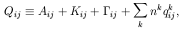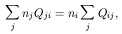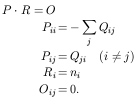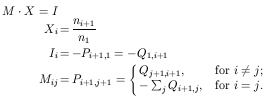2.3. Solving the system of statistical equilibrium equations

Let us now proceed to set the system of equations to calculate the relative population ratios.

For notation purposes, let us define:(15)

so that eq. (2) reads (taking into account the possible effect of fluorescence):(16)

or equivalently in matrix notation:(17)

After rewriting the system to have the solution expressed in terms of the populations relative to the ground level n1 we are left with:(18)

Eq. (18) is the linear system of equations that is solved by PopRatio.